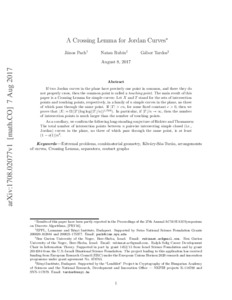REAL

# A Crossing Lemma for Jordan curves

Pach, János and Rubin, Natan and Tardos, Gábor (2018) A Crossing Lemma for Jordan curves. ADVANCES IN MATHEMATICS, 331. pp. 908-940. ISSN 0001-8708Preview
Text
1708.02077v1.pdf

Download (515kB) | Preview

## Abstract

If two Jordan curves in the plane have precisely one point in common, and there they do not properly cross, then the common point is called a touching point. The main result of this paper is a Crossing Lemma for simple curves: Let X and T stand for the sets of intersection points and touching points, respectively, in a family of n simple curves in the plane, no three of which pass through the same point. If |T|>cn, for some fixed constant c>0, then we prove that |X|=Ω(|T|(log⁡log⁡(|T|/n))1/504). In particular, if |T|/n→∞ then the number of intersection points is much larger than the number of touching points. As a corollary, we confirm the following long-standing conjecture of Richter and Thomassen: The total number of intersection points between n pairwise intersecting simple closed (i.e., Jordan) curves in the plane, no three of which pass through the same point, is at least (1−o(1))n2. © 2018

Item Type: Article Separators; Extremal problems; Crossing lemma; Contact graphs; Combinatorial geometry; Arrangements of curves Q Science / természettudomány > QA Mathematics / matematikaQ Science / természettudomány > QA Mathematics / matematika > QA166-QA166.245 Graphs theory / gráfelméletQ Science / természettudomány > QA Mathematics / matematika > QA73 Geometry / geometria MTMT SWORD MTMT SWORD 16 Aug 2018 13:58 16 Aug 2018 13:58 http://real.mtak.hu/id/eprint/82752

### Actions (login required)Edit Item